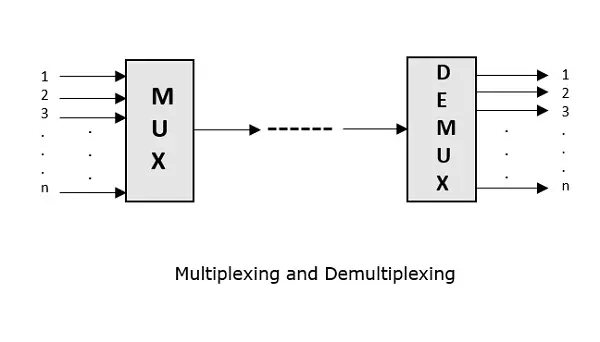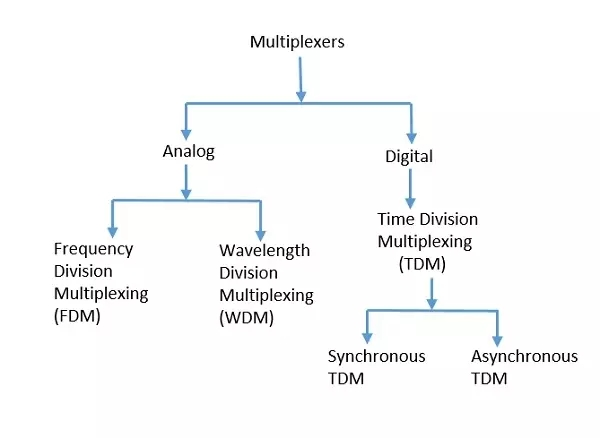# What is Multiplexing and what are its types?

Multiplexing is the process of combining multiple signals into one signal, over a shared medium. If analog signals are multiplexed, it is Analog Multiplexing and if digital signals are multiplexed, that process is Digital Multiplexing.The process of multiplexing divides a communication channel into several number of logical channels, allotting each one for a different message signal or a data stream to be transferred. The device that does multiplexing can be simply called as a MUX while the one that reverses the process which is demultiplexing, is called as DEMUX.

## Types of Multiplexers

There are mainly two types of multiplexers, namely analog and digital. They are further divided into FDM, WDM, and TDM.## Analog Multiplexing

The analog multiplexing techniques involve signals which are analog in nature. The analog signals are multiplexed according to their frequency (FDM) or wavelength (WDM).

Frequency Division Multiplexing (FDM)

In analog multiplexing, the most used technique is Frequency Division Multiplexing FDM. This technique uses various frequencies to combine streams of data, for sending them on a communication medium, as a single signal.

Example: A traditional television transmitter, which sends a number of channels through a single cable, uses FDM.

Wavelength Division Multiplexing (WDM)

Wavelength Division Multiplexing is an analog technique, in which many data streams of different wavelengths are transmitted in the light spectrum. If the wavelength increases, the frequency of the signal decreases.

Example: Optical fibre Communications use the WDM technique, to merge different wavelengths into a single light for the communication.

## Digital Multiplexing

The term digital represents the discrete bits of information. Hence the available data is in the form of frames or packets, which are discrete.

Time Division Multiplexing (TDM)

In TDM, the time frame is divided into slots. This technique is used to transmit a signal over a single communication channel, with allotting one slot for each message. Of all the types of TDM, the main ones are Synchronous and Asynchronous TDM.

Synchronous TDM

In Synchronous TDM, the input is connected to a frame. If there are ‘n’ number of connections, then the frame is divided into ‘n’ time slots. One slot is allocated for each input line. In this technique, the sampling rate is common to all signals and hence same clock input is given. The mux allocates the same slot to each device at all times.

Asynchronous TDM

In Asynchronous TDM, the sampling rate is different for each of the signals and the clock signal is also not in common. If the allotted device, for a time-slot, transmits nothing and sits idle, then that slot is allotted to another device, unlike synchronous.